Courses

# Chemistry - 2019 Past Year Paper

## 60 Questions MCQ Test IIT JAM Chemistry Past Year Papers | Chemistry - 2019 Past Year Paper

Description
This mock test of Chemistry - 2019 Past Year Paper for IIT JAM helps you for every IIT JAM entrance exam. This contains 60 Multiple Choice Questions for IIT JAM Chemistry - 2019 Past Year Paper (mcq) to study with solutions a complete question bank. The solved questions answers in this Chemistry - 2019 Past Year Paper quiz give you a good mix of easy questions and tough questions. IIT JAM students definitely take this Chemistry - 2019 Past Year Paper exercise for a better result in the exam. You can find other Chemistry - 2019 Past Year Paper extra questions, long questions & short questions for IIT JAM on EduRev as well by searching above.
QUESTION: 1

### For a reaction of the type A + B → Products, the unit of the rate constant is mol L–1 s–1. The overall order of the reaction is ______.

Solution:

Unit of k = (mole/L)1-n s-1
On Substituting, n=0
⇒ The unit of k = mol L–1 s–1
i.e. same as given.

Thus, the given reaction is a zero-order reaction or the overall order of the reaction is 0.

QUESTION: 2

### The thermodynamic criterion for spontaneity of a process in a system under constant volume and temperature and in the absence of any work other than expansion work (if any) is:

Solution: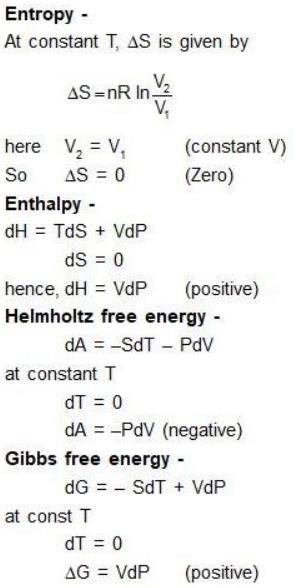QUESTION: 3

### The number of vibrational mode(s) of a carbon dioxide molecule that can be detected using infrared spectroscopy is ______.

Solution:
• CO2 molecule is linear so its vibrational degree of freedom is (3n - 5)
= (3 * 3 - 5) = 4
• 1 is symmetrical stretching means IR inactive and 3 is (symmetrical +bending) means IR active.
• Hence, the number of vibrational mode(s) of a carbon dioxide molecule that can be detected using infrared spectroscopy is 3.
QUESTION: 4

For three non-coplanar vectors a, b and c, the expression a ∙ (b × c) can be written as:

Solution:

(b×c) =b. (c×a) =-c.(a×b)=(a×b).c

So, (A) is the correct option.

QUESTION: 5

The correct trend in the bond order is:

Solution:

Bond order for the following:
(i) O2​ = 1.5
(ii) O2+​ = 2.5
(iii) O22- = 1

The correct trend will be: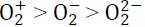QUESTION: 6

The correct option for the metal ion present in the active site of myoglobin, hemocyanin, and vitamin B12, respectively, are:

Solution: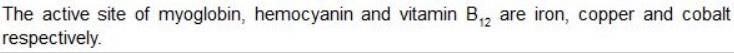QUESTION: 7

The correct order of wavelength (λmax) of the halide to metal charge-transfer band of [Co(NH3)5Cl]2+ (I), [Co(NH3)5Br]2+ (II) and [Co(NH3)5I]2+ (III), is

Solution:
• Down the group, Electronegativity decreases from Cl to I.
• So, in III less energy is required for the electron in Iodine to transfer, than that of Chlorine.
• As energy decreases, wavelength(λmax) increases from Cl to I.
QUESTION: 8

The correct option for the major products of the following reaction is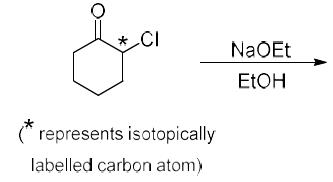Solution:

This is the reaction named by favorskii rearrangement in which base abstracts the proton from left carbon of carbonyl group and withdraws the leaving group Cl by rearrangement and makes ester.

QUESTION: 9

The major product formed in the following reaction is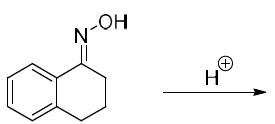Solution:
QUESTION: 10

The complementary strand for the following single strand of DNA is: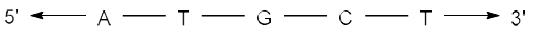Solution:
• In DNA, Adenine (A) pairs with Thymine (T) and Guanine (G) pairs with Cytosine (C).
• Hence, on replication of DNA in M phase, complementary nucleotides for each base pair will be added as follows:
(i) A - T
(ii) T - A
(iii) G - C
(iv) C - G
• Since replication occurs in 5'-3' direction, so the complementary strand will be formed in 3'-5' direction.
• Hence the sequence of complementary strand formed upon replication will be: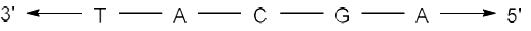QUESTION: 11

The function f(x) x e-x2 has a minimum at

Solution:
QUESTION: 12

The correct option for the number of bending modes of vibration in each of H2O, CS2 and SO2 molecules, respectively, is

Solution:
QUESTION: 13

The total number of degrees of freedom of an HBr molecule that is constrained to translate along a straight line but does not have any constraints for its rotation and vibration is:

Solution:
• The total degree of freedom of a molecule is 3N where N refers to its atomicity.
• Ideally, it should be 6 (∵ HBr is a diatomic linear molecule).
• But it is constrained to move along a straight line (i.e. assuming that it can move only along one axis).
• Therefore, the total translational degree of freedom would be 1.
• Hence, overall it would be 4.
QUESTION: 14

According to the kinetic theory of gases, the ratio of the root mean square velocity of molecular oxygen and molecular hydrogen at 300 K is:

Solution:

Vrmsoxygen/Vrmshydrogen = √Mhydrogen/√Moxygen
∵ Vrms is inversely proportional to the molar mass

Vrmsoxygen/Vrmshydrogen = √1/√16 = 1/4

QUESTION: 15

The half-life of the chemical reaction, A → Product, for initial reactant concentrations of 0.1 and 0.4 mol L–1 are 200 and 50 s, respectively. The order of the reaction is:

Solution: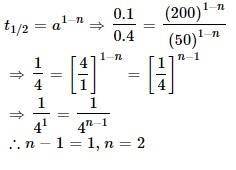QUESTION: 16

The ratio of the nearest neighbor atomic distances in body-centered cubic (bcc) and facecentered cubic (fcc) crystals with the same unit cell edge length is

Solution:
• In BCC, the nearest atom from one corner is at the body center, at a distance of √(3a/2).
• In FCC, the nearest atom from one corner is at the face center at a distance of √(2a/2).
• The ratio would be √(3/2).
QUESTION: 17

The correct trend in the rate of substitution of Cl- by pyridine in the following complexes is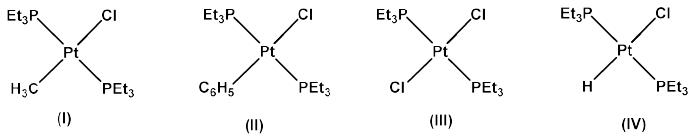Solution:
QUESTION: 18

In the qualitative inorganic analysis of metal ions, the ion which precipitates as sulfide in the presence of H2S in warm dilute HCl is:

Solution:
• Group II → Bi3+
Group III A → Al3+, Cr3+
Group III B → Co2+
• Second group cations are precipitated as sulphides because of their low solubility products whereas sulphides of other metals remain in solution because of their high solubility products.
• HCl acts as a source of H+ which decrease the concentration of S2− ion that exceeds only the solubility products of the metal sulphide of the IInd group.
QUESTION: 19

The correct statement regarding the observed magnetic properties of NO, O2, B2, and C2 in their ground state is:

Solution:
• In B2, there are two unpaired electrons in the π bonding orbital.
• In O2, there are also two π electrons in antibonding orbitals.
• In NO, there is one unpaired electron in π* antibonding orbital
• Since the paramagnetic nature is based on the presence of unpaired electrons.
• Thus, B2, O2, and NO are paramagnetic.
QUESTION: 20

The observed magnetic moments of octahedral Mn3+, Fe3+ and Co3+ complexes are 4.95, 6.06 and 0.00 BM, respectively. The correct option for the electronic configuration of Mn3+, Fe3+ and Co3+ metal ions in these complexes, respectively, is

Solution:
QUESTION: 21

Among the following compounds, the one having the lowest boiling point is

Solution:
QUESTION: 22

The correct option having one complex from each of the following pairs which is more reactive towards the oxidative addition reaction by hydrogen molecule is
Pair 1: IrCl(PMe3)3 (I) and IrCl(CO)(PMe3)2 (II)
Pair 2: IrCl(CO)(PPh3)2 (III) and IrCl3(PPh3) (IV)

Solution:
QUESTION: 23

Among the following, the correct statement is

Solution:
QUESTION: 24

The major product of the following reaction is: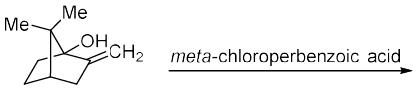Solution:
QUESTION: 25

In 1H NMR spectrum of the given molecule, the correct order of chemical shifts of the labelled protons (HX, HY, HZ) is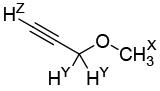Solution:
QUESTION: 26

In the following reaction of (D)-Glucose, a product P is formed.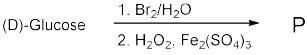Among the following compounds, the one which will give the same product (P) under identical reaction conditions is

Solution:
QUESTION: 27

The major product of the following reaction is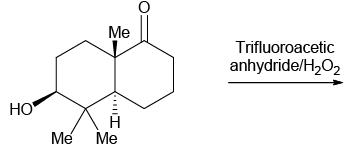Solution:
QUESTION: 28

The correct option for the product(s) of the following reaction is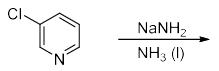Solution:
QUESTION: 29

The increasing order of acidity of the given molecules in aqueous media is: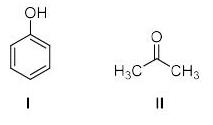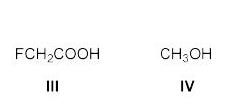Solution: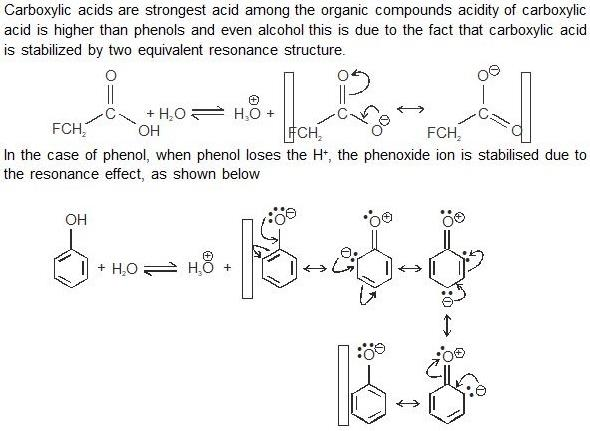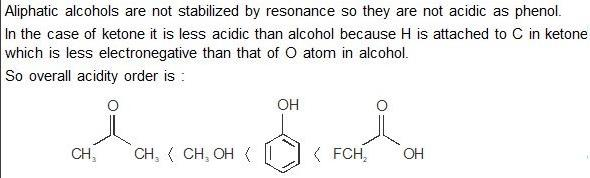QUESTION: 30

The compound formed upon subjecting an aliphatic amine to Lassaigne’s test is

Solution: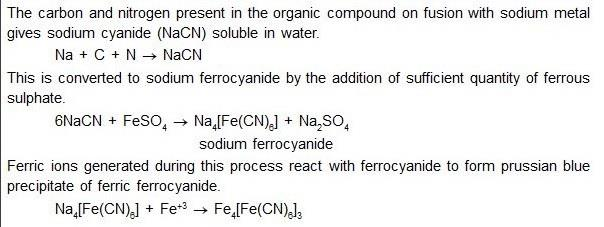*Multiple options can be correct
QUESTION: 31

The eigenvalue(s) of the matrix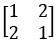is/are:

Solution:

Product of Eigenvalues = det A
Sum of Eigenvalues = trace A

Let a,b be the Eigenvalues of A.
Therefore,
a+b = 2
a.b = -3
On solving the two equations,
We get -1 and 3

*Multiple options can be correct
QUESTION: 32

The unit of the constant ‘a’ in van der Waals equation of state of a real gas can be expressed as

Solution:
*Multiple options can be correct
QUESTION: 33

Among the following, microwave active molecule(s) is/are​:

Solution:
• Microwave active molecules are those molecules that have permanent dipoles.
• If we look at the structure of trans-dichloro-ethene, it has dipole moment 0 due to Cl attached opposite side.
• Whereas all others are polar draw the structures.
*Multiple options can be correct
QUESTION: 34

The true statement(s) regarding the brown ring test carried out in the laboratory for the detection of NO3- is/are

Solution:
• Complex formed is [Fe(H2O)5NO]SO4.
• Concentrated Sulphuric acid is used.
• Brown ring is formed at the junction of two layers.
• Brown coloured complex is paramagnetic.
*Multiple options can be correct
QUESTION: 35

The true statement(s) regarding the carbonic anhydrase enzyme is/are

Solution:
*Multiple options can be correct
QUESTION: 36

The correct statement(s) about NO2, NO2+ and CO2 is /are:

Solution:
• NO2→ sp hybridization, linear, no unpaired electrons, Diamagnetic.
• NO2 → sp2 hybridization, V-shape, one unpaired electron, Paramagnetic.
• CO2 → sp hybridization, linear, no unpaired electrons, Diamagnetic.
• COand NO2+ have 22 e- each, therefore they are isoelectronic.
*Multiple options can be correct
QUESTION: 37

The compound(s) formed as intermediate(s) in the following reaction sequence is/are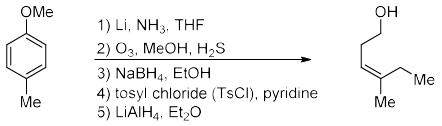Solution:
*Multiple options can be correct
QUESTION: 38

The correct statement(s) among the following is/are

Solution:
*Multiple options can be correct
QUESTION: 39

The diastereomeric pair(s) among the following option(s) is/are

Solution:
*Multiple options can be correct
QUESTION: 40

The reaction(s) that result(s) in the formation of aromatic species is/are

Solution:
*Answer can only contain numeric values
QUESTION: 41

The bond order of N2+ ion is __________. (Round off to one decimal place)

Solution:

No of bonding electrons = 9
No of antibonding electrons = 4
Bond Order = (1/2) (9 - 4) = 5/2 = 2.5

*Answer can only contain numeric values
QUESTION: 42

One liter of a buffer solution contains 0.004 mole of acetic acid (pKa = 4.76) and 0.4 mole of sodium acetate. The pH of the solution is __________. (Round off to two decimal places)

Solution:
*Answer can only contain numeric values
QUESTION: 43

The limiting molar conductivity of La3+ and Cl ions in aqueous medium at 298 K are 209.10 x10–4 and 76.35 x 10–4 S m2 mol–1, respectively. The transport number of Cl in an infinitely dilute aqueous solution of LaCl3 at 298 K is __________. (Round off to two decimal places)

Solution:

In terms of concentration t- = molar conductance of cation ÷ total molar conductance
Since there are three Cl- ions = 3× (76.35)
= 229.05
total molar conductance = 1× 209.10 + 3× ( 76.35)
= 438 .15
so. = 229.05÷ 438.15
=0.5227

*Answer can only contain numeric values
QUESTION: 44

The magnetic field strength required to excite an isolated proton to its higher spin state with an electromagnetic radiation of 300 MHz is __________ Tesla (T). (Round off to two decimal places)
[Magnetogyric ratio of proton is 26.75 x 107 rad T–1 s–1]

Solution:
*Answer can only contain numeric values
QUESTION: 45

The value of n for the complex [Fe(CO)4(SiMe3)]n satisfying the 18-electron rule is __________.

Solution:
*Answer can only contain numeric values
QUESTION: 46

In the structure of P4O10, the number of P-O-P bond(s) is __________.

Solution:
*Answer can only contain numeric values
QUESTION: 47

Number of vertices in an icosahedral closo-borane is __________.

Solution:
*Answer can only contain numeric values
QUESTION: 48

Based on the information given below, the isoelectric point (pI) of lysine is __________. (Round off to one decimal place)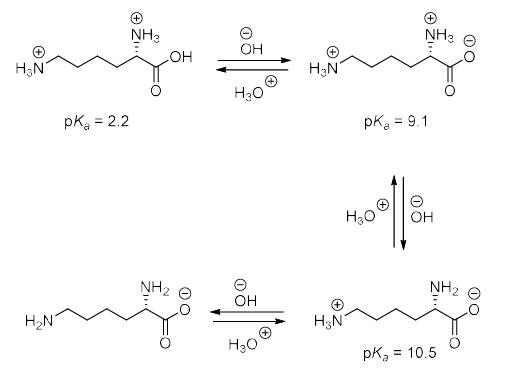Solution:

Here pka1 is 9.1
pka2 is 10.5
so the isoelectronic point of lysine is (9.1+10.5)/2=9.8

*Answer can only contain numeric values
QUESTION: 49

(R)-2-methyl-1-butanol has a specific rotation of +13.5°. The specific rotation of 2-methyl1-butanol containing 40% of the (S)-enantiomer is __________ °. (Round off to one decimal place)

Solution:
*Answer can only contain numeric values
QUESTION: 50

The number of gauche-butane interaction(s) in the following compound is__________.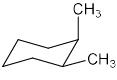Solution:
*Answer can only contain numeric values
QUESTION: 51

The ionization energy of hydrogen atom is 13.6 eV and the first ionization energy of sodium atom is 5.1 eV. The effective nuclear charge experienced by the valance electron of sodium atom is __________. (Round off to one decimal place)

Solution:
*Answer can only contain numeric values
QUESTION: 52

One mole of an ideal gas is subjected to an isothermal increase in pressure from 100 kPa to 1000 kPa at 300 K. The change in Gibbs free energy of the system is __________ kJ mol–1.
(Round off to one decimal place)
[Given: Gas constant (R) = 8.3 J K–1 mol–1]

Solution:
*Answer can only contain numeric values
QUESTION: 53

One liter of an aqueous urea solution contains 6 g of urea. The osmotic pressure of the solution at 300 K (assuming an ideal behavior) is __________ kPa. (Round off to one decimal place)
[Given: Molecular weight of urea is 60, gas constant (R) is 8.3 J K–1 mol–1]

Solution:
*Answer can only contain numeric values
QUESTION: 54

A first order reflection of X-ray from {220} plane of copper crystal is observed at a glancing angle of 22°. The wavelength of the X-ray used is __________ pm. (Round off to one decimal place)
[Given: Copper forms fcc crystal with unit cell edge length of 361 pm.]

Solution:
*Answer can only contain numeric values
QUESTION: 55

The collision flux of a monoatomic gas on copper surface is 3.0 x 1018 m–2  s–1. Note that copper surface forms a square lattice with lattice constant of 210 pm. If the sticking coefficient of the atom with copper is 1.0, the time taken by the gas to form a complete monolayer on the surface is __________ s. (Round off to one decimal place)

Solution:
*Answer can only contain numeric values
QUESTION: 56

The turnover frequency (TOF) for the catalytic reaction,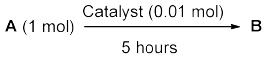with 90% yield of the product is __________ hour-1. (Round off to the nearest integer)

Solution:
*Answer can only contain numeric values
QUESTION: 57

A radioactive sample decays to 10% of its initial amount in 4600 minutes. The rate constant of this process is __________ hour-1. (Round off to two decimal places)

Solution:
*Answer can only contain numeric values
QUESTION: 58

Given that the radius of the first Bohr orbit of hydrogen atom is 53 pm, the radius of its third Bohr orbit is __________ pm. (Round off to the nearest integer)

Solution:
*Answer can only contain numeric values
QUESTION: 59

5.3 g of benzaldehyde was reacted with an excess of acetophenone to produce 5.2 g of the enone product as per the reaction shown below. The yield of the reaction is __________ %. (Round off to the nearest integer)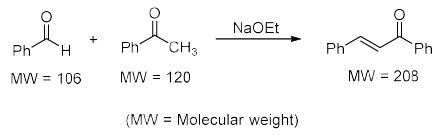Solution:
*Answer can only contain numeric values
QUESTION: 60

Assume that the reaction of MeMgBr with ethylacetate proceeds with 100% conversion to give tert-butanol. The volume of 0.2 M solution of MeMgBr required to convert 10 mL of a 0.025 M solution of ethylacetate to tert-butanol is __________ mL. (Round off to one decimal place)

Solution: